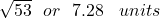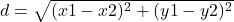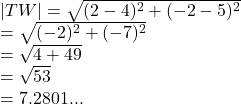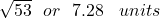## What is the distance between the points? * T(2,-2) W(4,5)

Question

What is the distance between the points?
*
T(2,-2)
W(4,5)

in progress 0
2 months 2021-07-23T00:27:22+00:00 1 Answers 4 views 0

##Step-by-step explanation:

The distance between two points can be found by using the formulawhere

(x1 , y1) and (x2 , y2) are the points

From the question the points are

T(2,-2) , W(4,5)

The distance between them isWe have the final answer asHope this helps you